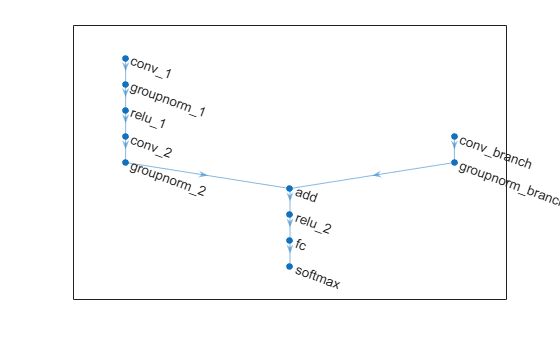# initialize

Initialize learnable and state parameters of a `dlnetwork`

Since R2021a

## Syntax

``netUpdated = initialize(net)``
``netUpdated = initialize(net,X1,...,Xn)``

## Description

example

``` TipMost `dlnetwork` objects are initialized by default. You only need to manually initialize a `dlnetwork` if it is uninitialized. You can check if a network is initialized using the `Initialized` property of the `dlnetwork` object. `netUpdated = initialize(net)` initializes any unset learnable parameters and state values of `net` based on the input sizes defined by the network input layers. Any learnable or state parameters that already contain values remain unchanged. A network with unset, empty values for learnable and state parameters is uninitialized. You must initialize an uninitialized `dlnetwork` before you can use it. By default, `dlnetwork` objects are constructed with initial parameters and do not need initializing. ```

example

````netUpdated = initialize(net,X1,...,Xn)` initializes any unset learnable parameters and state values of `net` based on the example network inputs or network data layout objects `X1,...,Xn`. Use this syntax when the network has inputs that are not connected to an input layer.```

## Examples

collapse all

Define a simple image classification network as a layer array.

```layers = [ imageInputLayer([28 28 1],Normalization="none") convolution2dLayer(5,20) batchNormalizationLayer reluLayer fullyConnectedLayer(10) softmaxLayer];```

Convert the layer graph to a `dlnetwork` object. Create an uninitialized `dlnetwork` object by setting the `Initialize` option to `false`.

`net = dlnetwork(layers,Initialize=false);`

View the learnable parameters of the network. Because the network is not initialized, the values are empty.

`net.Learnables`
```ans=6×3 table Layer Parameter Value ___________ _________ ____________ "conv" "Weights" {0x0 double} "conv" "Bias" {0x0 double} "batchnorm" "Offset" {0x0 double} "batchnorm" "Scale" {0x0 double} "fc" "Weights" {0x0 double} "fc" "Bias" {0x0 double} ```

Initialize the learnable parameters of the network using the `initialize` function.

`net = initialize(net);`

View the learnable parameters of the network. Because the network is now initialized, the values are nonempty with sizes inferred using the size of the input layer.

`net.Learnables`
```ans=6×3 table Layer Parameter Value ___________ _________ ___________________ "conv" "Weights" { 5x5x1x20 dlarray} "conv" "Bias" { 1x1x20 dlarray} "batchnorm" "Offset" { 1x1x20 dlarray} "batchnorm" "Scale" { 1x1x20 dlarray} "fc" "Weights" {10x11520 dlarray} "fc" "Bias" {10x1 dlarray} ```

Define a multi-input image classification network.

```numFilters = 24; layersBranch1 = [ convolution2dLayer(3,6*numFilters,Padding="same",Stride=2) groupNormalizationLayer("all-channels") reluLayer convolution2dLayer(3,numFilters,Padding="same") groupNormalizationLayer("channel-wise") additionLayer(2,Name="add") reluLayer fullyConnectedLayer(10) softmaxLayer]; layersBranch2 = [ convolution2dLayer(1,numFilters,Name="conv_branch") groupNormalizationLayer("all-channels",Name="groupnorm_branch")]; lgraph = layerGraph(layersBranch1); lgraph = addLayers(lgraph,layersBranch2); lgraph = connectLayers(lgraph,"groupnorm_branch","add/in2"); ```

Visualize the layer graph in a plot.

```figure plot(lgraph)```Convert the layer graph to a `dlnetwork` object. Create an uninitialized `dlnetwork` object by setting the `Initialize` option to `false`.

`net = dlnetwork(lgraph,Initialize=false);`

View the learnable parameters of the network. Because the network is not initialized, the values are empty.

`net.Learnables`
```ans=14×3 table Layer Parameter Value __________________ _________ ____________ "conv_1" "Weights" {0x0 double} "conv_1" "Bias" {0x0 double} "groupnorm_1" "Offset" {0x0 double} "groupnorm_1" "Scale" {0x0 double} "conv_2" "Weights" {0x0 double} "conv_2" "Bias" {0x0 double} "groupnorm_2" "Offset" {0x0 double} "groupnorm_2" "Scale" {0x0 double} "fc" "Weights" {0x0 double} "fc" "Bias" {0x0 double} "conv_branch" "Weights" {0x0 double} "conv_branch" "Bias" {0x0 double} "groupnorm_branch" "Offset" {0x0 double} "groupnorm_branch" "Scale" {0x0 double} ```

View the names of the network inputs.

`net.InputNames`
```ans = 1x2 cell {'conv_1'} {'conv_branch'} ```

Create random `dlarray` objects representing inputs to the network. Use an example input of size 64-by-64 with 3 channels for the main branch of the network. Use an input of size 64-by-64 with 18 channels for the second branch.

```inputSize = [64 64 3]; inputSizeBranch = [32 32 18]; X1 = dlarray(rand(inputSize),"SSCB"); X2 = dlarray(rand(inputSizeBranch),"SSCB");```

Initialize the learnable parameters of the network using the `initialize` function and specify the example inputs. Specify the inputs with order corresponding to the `InputNames` property of the network.

`net = initialize(net,X1,X2);`

View the learnable parameters of the network. Because the network is now initialized, the values are nonempty with sizes inferred using the size of the input data.

`net.Learnables`
```ans=14×3 table Layer Parameter Value __________________ _________ _____________________ "conv_1" "Weights" { 3x3x3x144 dlarray} "conv_1" "Bias" { 1x1x144 dlarray} "groupnorm_1" "Offset" { 1x1x144 dlarray} "groupnorm_1" "Scale" { 1x1x144 dlarray} "conv_2" "Weights" { 3x3x144x24 dlarray} "conv_2" "Bias" { 1x1x24 dlarray} "groupnorm_2" "Offset" { 1x1x24 dlarray} "groupnorm_2" "Scale" { 1x1x24 dlarray} "fc" "Weights" { 1x1x18x24 dlarray} "fc" "Bias" { 1x1x24 dlarray} "conv_branch" "Weights" { 1x1x24 dlarray} "conv_branch" "Bias" { 1x1x24 dlarray} "groupnorm_branch" "Offset" {10x24576 dlarray} "groupnorm_branch" "Scale" {10x1 dlarray} ```

Create an uninitialized `dlnetwork` object that has two unconnected inputs.

```layers = [ convolution2dLayer(5,16,Name="conv") batchNormalizationLayer reluLayer fullyConnectedLayer(50) flattenLayer concatenationLayer(1,2,Name="cat") fullyConnectedLayer(10) softmaxLayer]; net = dlnetwork(layers,Initialize=false);```

View the input names of the network.

`net.InputNames`
```ans = 1×2 cell {'conv'} {'cat/in2'} ```

Create network data layout objects that represent input data for the inputs. For the first input, specify a batch of 28-by-28 grayscale images. For the second input specify a batch of single-channel feature data.

```layout1 = networkDataLayout([28 28 1 NaN],"SSCB"); layout2 = networkDataLayout([1 NaN],"CB");```

Initialize the network using the network data layout objects.

`net = initialize(net,layout1,layout2)`
```net = dlnetwork with properties: Layers: [8×1 nnet.cnn.layer.Layer] Connections: [7×2 table] Learnables: [8×3 table] State: [2×3 table] InputNames: {'conv' 'cat/in2'} OutputNames: {'softmax'} Initialized: 1 View summary with summary. ```

## Input Arguments

collapse all

Uninitialized network, specified as a `dlnetwork` object.

Example network inputs or data layouts, specified as formatted `dlarray` objects or formatted `networkDataLayout` objects. The software propagates `X1,...Xn` through the network to determine the appropriate sizes and formats of the learnable and state parameters of the `dlnetwork`.

Provide example inputs in the same order as the order specified by the `InputNames` property of the input network.

Note

Automatic initialization uses only the size and format information of the input data. For initialization that depends on the values on the input data, you must initialize the learnable parameters manually.

## Output Arguments

collapse all

Initialized network, returned as an initialized `dlnetwork` object.

The `initialize` function does not preserve quantization information. If the input network is a quantized network, then the output network does not contain quantization information.

## Version History

Introduced in R2021a